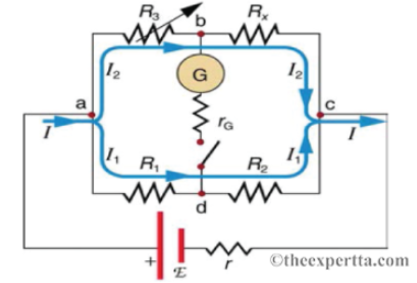# Problem: Consider a Wheatstone bridge with some known resistances: Rx is 95 Ω, R1 is 25 Ω, and R2 is 185 Ω.To what value, in ohms, must you adjust R3 to balance the Wheatstone bridge?R3 =

###### FREE Expert Solution

Ohms law,

$\overline{){\mathbf{V}}{\mathbf{=}}{\mathbf{i}}{\mathbf{R}}}$

In a balanced state,

i2R3 = i1R1

i2/i1 = R1/R3

Vbc = Vdc

94% (62 ratings)###### Problem Details

Consider a Wheatstone bridge with some known resistances: Rx is 95 Ω, R1 is 25 Ω, and R2 is 185 Ω.To what value, in ohms, must you adjust R3 to balance the Wheatstone bridge?

R3